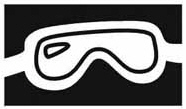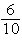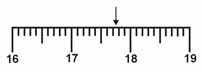Name:    Unit 1 Quiz Practice

Multiple Choice
Identify the choice that best completes the statement or answers the question.

1.

Which precaution should you take when you see this symbol?a. do not look directly at the experiment b. wear goggles if you are doing the experiment c. wear goggles if you are performing or observing the experiment d. watch the teacher carefully and follow what he or she does

2.

Which of the following is qualitative data?
 a. Color c. Temperature b. Pressure d. Volume

3.

Which of the following is quantitative data?
 a. Color c. Shape b. Odor d. Volume

4.

A theory is a ____.
 a. proposed explanation for an observation b. well-tested explanation for a broad set of observations c. summary of the results of many observations d. procedure used to test a proposed explanation

5.

Which of the following is an example of a safe laboratory procedure?
 a. tying back long hair and loose clothing b. eating or drinking from laboratory glassware c. touching hot objects with your bare hands d. testing an odor by directly inhaling the vapor

6.

How is 0.00069 written in scientific notation?
 a. 69 ´ 10–5 c. 0.69 ´ 10–3 b. 6.9 ´ 104 d. 6.9 ´ 10–4

7.

Which of the following conversion factors would you use to change 18 kilometers to meters?
 a. 1000 m/1 km c. 100 m/1 km b. 1 km/1000 m d. 1 km/100 m

8.

What are 6 centimeters equal to?
 a. 600 meters c. 60 millimeters b.of a millimeter d. 600 millimeters

9.

Which is the correct measurement for location marked by the arrow?a. 17.076 c. 17.76 b. 17.8 d. 17.7

10.

The accepted value is 1.43. Which correctly describes this student’s experimental data?
 Trial Measurement 1 1.29 2 1.93 3 0.88
 a. accurate but not precise c. both accurate and precise b. precise but not accurate d. neither accurate nor precise

11.

Which term is described as the amount of matter in an object?
 a. density c. mass b. volume d. length

12.

All of the following are examples of units except
 a. mass. c. gram. b. kilometer. d. ounce.

13.

The symbols for units of length in order from largest to smallest are
 a. m, cm, mm, km. c. km, mm, cm, m. b. mm, m, cm, km. d. km, m, cm, mm.

14.

Which of these metric units is used to measure mass?
 a. m c. g b. mm d. L

15.

The quantity of matter per unit volume is
 a. mass. c. inertia. b. weight. d. density.

16.

A change in the force of gravity on an object will affect its
 a. mass. c. weight. b. density. d. kinetic energy.

17.

Which pair of quantities determines the density of a material?
 a. mass and weight c. volume and concentration b. volume and weight d. volume and mass

18.

The density of an object is calculated by
 a. multiplying its mass times its volume. b. dividing its mass by its volume. c. dividing its volume by its mass. d. adding its mass to its volume.

19.

0.25 g is equivalent to
 a. 250 kg. c. 0.025 mg. b. 250 mg. d. 0.025 kg.

20.

The number of grams equal to 0.5 kg is
 a. 0.0005. c. 500 b. 0.005. d. 5000

21.

These values were recorded as the mass of products when a chemical reaction was carried out three separate times: 8.83 g; 8.84 g; 8.82 g. The mass of products from that reaction is
8.60 g. The values are
 a. accurate, but not precise. b. precise, but not accurate. c. both accurate and precise. d. neither accurate nor precise.

22.

How is the measurement 0.000 065 cm written in scientific notation?
 a. 65 ´ 10–6 cm c. 6.5 ´ 10–6 cm b. 6.5 ´ 10–5 cm d. 6.5 ´ 10–4 cm

23.

The measurement 0.020 L is the same as
 a. 2.0 ´ 10–3 L. c. 2.0 ´ 10–2 L. b. 2.0 ´ 102 L. d. 2.0 ´ 10–1 L.

24.

How would 0.00930 m be expressed in scientific notation?
 a. 93 ´ 10–4 m c. 9.30 ´ 10–3 m b. 9.3 ´ 10–4 m d. 9.30 ´ 10–5 m

25.

When 1.92 ´ 10–6 kg is divided by 6.8 ´ 102 mL, the quotient equals
 a. 2.8 ´ 10–4 kg/mL. c. 2.8 ´ 10–8 kg/mL. b. 2.8 ´ 10–5 kg/mL. d. 2.8 ´ 10–9 kg/mL.

26.

When 6.02 ´ 1023 is multiplied by 9.1 ´ 10–31, the product is
 a. 4.3 ´ 10–8. c. 4.3 ´ 10–7. b. 4.3 ´ 1054. d. 4.3 ´ 10–53.

27.

The result of dividing 107 by 10–3 is
 a. 10–4. c. 104 b. 102.5. d. 1010

28.

The capacity of a Florence flask is 250 mL. Its capacity in liters expressed in scientific notation is
 a. 2.5 ´ 10–2 L. c. 2.5 ´ 101 L. b. 2.5 ´ 10–1 L. d. 2.5 ´ 102 L.